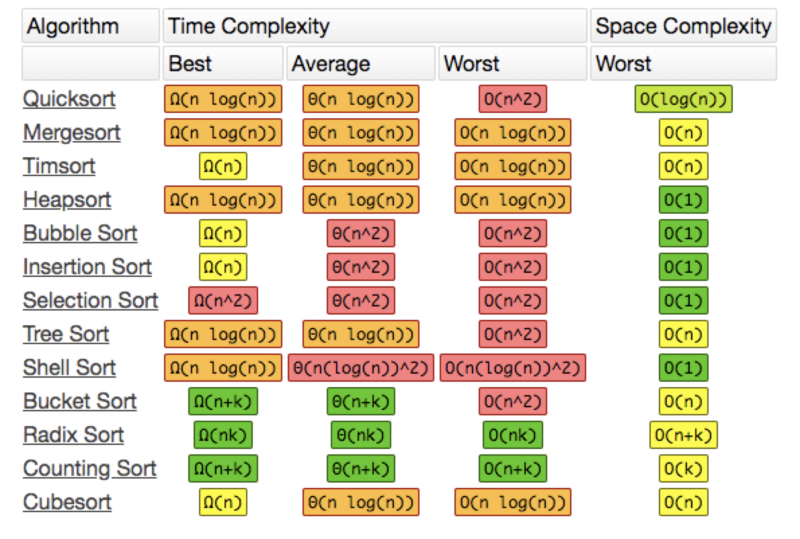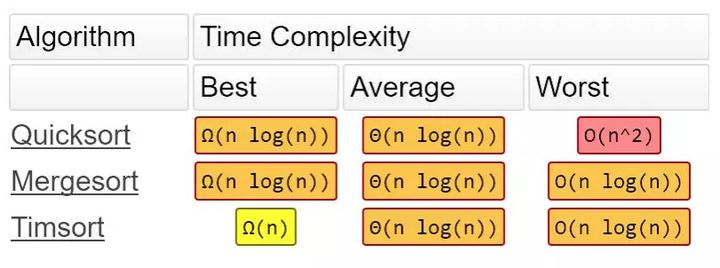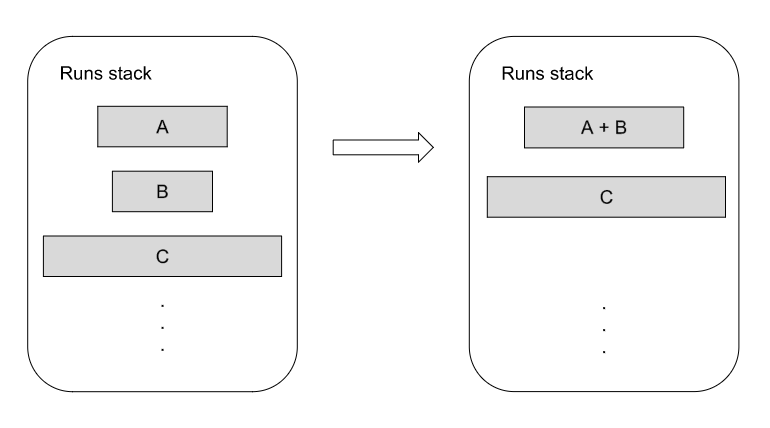# # 讲下 V8 sort 的大概思路，并手写一个 sort 的实现## # Array.prototype.sort()

`sort()` 方法用原地算法对数组的元素进行排序，并返回数组。默认排序顺序是在将元素转换为字符串，然后比较它们的UTF-16代码单元值序列时构建的

— MDN

``````const array = [1, 30, 4, 21, 100000];
array.sort();
console.log(array);
// [1, 100000, 21, 30, 4]

const numbers = [4, 2, 5, 1, 3];
numbers.sort((a, b) => a - b);
console.log(numbers)
// [1, 2, 3, 4, 5]
``````

## # V8 种的 Array.prototype.sort()## # 什么是 TimSort ？

Timsort 是 Tim Peter 在 2001 年为 Python 语言特意创造的，主要是 基于现实数据集中存在者大量的有序元素（不需要重新排序） 。 Timsort 会遍历所有数据，找出数据中所有有序的分区（run），然后按照一定的规则将这些分区（run）归并为一个。

• 扫描数组，并寻找所谓的 `_runs_` ，一个 run 可以认为是已经排序的小数组，也包括以逆向排序的，因为这些数组可以简单地翻转（reverse）就成为一个run
• 确定最小 run 长度，小于的 run 会通过 插入排序 归并成长度高于最小长度的 run
• 反复归并一些相邻 run ，过程中避免归并长度相差很大的片段，直至整个排序完成

• `|C| > |B| + |A|`
• `|B| > |A|`## # 手写一个 Array.prototype.sort() 实现

``````// 顺序合并两个小数组left、right 到 result
function merge(left, right) {
let result = [],
ileft = 0,
iright = 0
while(ileft < left.length && iright < right.length) {
if(left[ileft] < right[iright]){
result.push(left[ileft ++])
} else {
result.push(right[iright ++])
}
}
while(ileft < left.length) {
result.push(left[ileft ++])
}
while(iright < right.length) {
result.push(right[iright ++])
}
return result
}

// 插入排序
function insertionSort(arr) {
let n = arr.length;
let preIndex, current;
for (let i = 1; i < n; i++) {
preIndex = i - 1;
current = arr[i];
while (preIndex >= 0 && arr[preIndex] > current) {
arr[preIndex + 1] = arr[preIndex];
preIndex--;
}
arr[preIndex + 1] = current;
}
return arr;
}

// timsort
function timsort(arr) {
// 空数组或数组长度小于 2，直接返回
if(!arr || arr.length < 2) return arr
let runs = [],
sortedRuns = [],
newRun = [arr],
length = arr.length
// 划分 run 区，并存储到 runs 中，这里简单的按照升序划分，没有考虑降序的run
for(let i = 1; i < length; i++) {
if(arr[i] < arr[i - 1]) {
runs.push(newRun)
newRun = [arr[i]]
} else {
newRun.push(arr[i])
}
if(length - 1 === i) {
runs.push(newRun)
break
}
}
// 由于仅仅是升序的run，没有涉及到run的扩充和降序的run，因此，其实这里没有必要使用 insertionSort 来进行 run 自身的排序
for(let run of runs) {
insertionSort(run)
}
// 合并 runs
sortedRuns = []
for(let run of runs) {
sortedRuns = merge(sortedRuns, run)
}
return sortedRuns
}

// 测试
var numbers = [4, 2, 5, 1, 3]
timsort(numbers)
// [1, 2, 3, 4, 5]
``````

## # v8 中的 Array.prototype.sort() 源码解读

1. 判断数组长度，小于2直接返回，不排序
2. 开始循环
3. 找出一个有序子数组，我们称之为 “run” ，长度 currentRunLength
4. 计算最小合并序列长度 minRunLength （这个值会根据数组长度动态变化，在32~64之间）
5. 比较 currentRunLength 和 minRunLength ，如果 currentRunLength >= minRunLength ，否则采用插入排序补足数组长度至 minRunLength ，将 run 压入栈 pendingRuns 中
6. 每次有新的 run 被压入 pendingRuns 时保证栈内任意 3 个连续的 run（run0, run1, run2）从下至上满足run0 > run1 + run2 && run1 > run2 ，不满足的话进行调整直至满足
7. 如果剩余子数组为 0 ，结束循环
8. 合并栈中所有 run，排序结束

### # 核心源码解读

• `ComputeMinRunLength` ：用来计算 `minRunLength`
• `CountAndMakeRun` ：计算第一个 `run` 的长度
• `MergeCollapse` ：调整 `pendingRuns` ，使栈长度大于 `3` 时，所有 `run` 都满足 `run[n]>run[n+1]+run[n+2]``run[n+1]>run2[n+2]`
``````// 计算最小合并序列长度 minRunLength
macro ComputeMinRunLength(nArg: Smi): Smi {
let n: Smi = nArg;
let r: Smi = 0;  // Becomes 1 if any 1 bits are shifted off.

assert(n >= 0);
// 如果小于 64 ，则返回n（该值太小，无法打扰那些奇特的东西）
// 否则不断除以2，得到结果在 32~64 之间
while (n >= 64) {
r = r | (n & 1);
n = n >> 1;
}

const minRunLength: Smi = n + r;
assert(nArg < 64 || (32 <= minRunLength && minRunLength <= 64));
return minRunLength;
}
``````
``````// 计算第一个 run 的长度
macro CountAndMakeRun(implicit context: Context, sortState: SortState)(
lowArg: Smi, high: Smi): Smi {
assert(lowArg < high);
// 这里保存的才是我们传入的数组数据
const workArray = sortState.workArray;

const low: Smi = lowArg + 1;
if (low == high) return 1;

let runLength: Smi = 2;

const elementLow = UnsafeCast<JSAny>(workArray.objects[low]);
const elementLowPred = UnsafeCast<JSAny>(workArray.objects[low - 1]);
// 调用比对函数来比对数据
let order = sortState.Compare(elementLow, elementLowPred);

// TODO(szuend): Replace with "order < 0" once Torque supports it.
//               Currently the operator<(Number, Number) has return type
//               'never' and uses two labels to branch.
const isDescending: bool = order < 0 ? true : false;

let previousElement: JSAny = elementLow;
// 遍历子数组并计算 run 的长度
for (let idx: Smi = low + 1; idx < high; ++idx) {
const currentElement = UnsafeCast<JSAny>(workArray.objects[idx]);
order = sortState.Compare(currentElement, previousElement);

if (isDescending) {
if (order >= 0) break;
} else {
if (order < 0) break;
}

previousElement = currentElement;
++runLength;
}

if (isDescending) {
ReverseRange(workArray, lowArg, lowArg + runLength);
}

return runLength;
}
``````
``````// 调整 pendingRuns ，使栈长度大于3时，所有 run 都满足 run[n]>run[n+1]+run[n+2] 且 run[n+1]>run2[n+2]
transitioning macro MergeCollapse(context: Context, sortState: SortState) {
const pendingRuns: FixedArray = sortState.pendingRuns;

// Reload the stack size because MergeAt might change it.
while (GetPendingRunsSize(sortState) > 1) {
let n: Smi = GetPendingRunsSize(sortState) - 2;

if (!RunInvariantEstablished(pendingRuns, n + 1) ||
!RunInvariantEstablished(pendingRuns, n)) {
if (GetPendingRunLength(pendingRuns, n - 1) <
GetPendingRunLength(pendingRuns, n + 1)) {
--n;
}

MergeAt(n); // 将第 n 个 run 和第 n+1 个 run 进行合并
} else if (
GetPendingRunLength(pendingRuns, n) <=
GetPendingRunLength(pendingRuns, n + 1)) {
MergeAt(n); // 将第 n 个 run 和第 n+1 个 run 进行合并
} else {
break;
}
}
}
``````